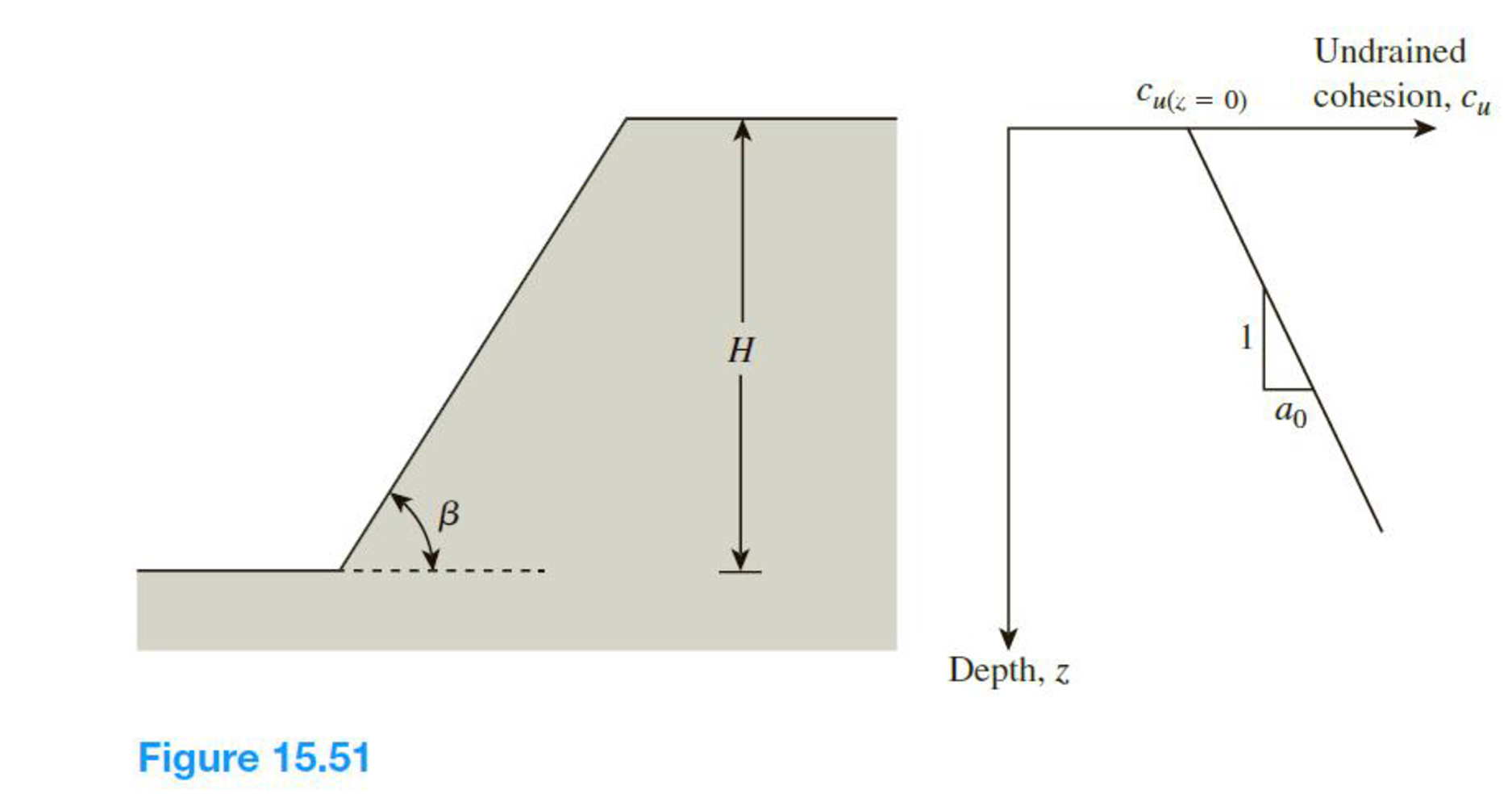Chapter 15, Problem 15.17PPrinciples of Geotechnical Enginee...

9th Edition
Braja M. Das + 1 other
ISBN: 9781305970939

Solutions

Chapter
SectionPrinciples of Geotechnical Enginee...

9th Edition
Braja M. Das + 1 other
ISBN: 9781305970939
Textbook Problem

A cut slope in saturated clay is inclined at an angle β = 26.57° (1 vertical to 2 horizontal) and has a height, H = 7 m. The undrained cohesion. cu, increases with depth, z, as shown in Figure 15.51. Assuming the critical circle to be a toe circle, determine the factor of safety, Fs using the Koppula (1984) method. Given: γsat= 18.6 kN/m3, cu(z = 0) = 9 kN/m2, and a0 = 5 kN/m3.To determine

Find the factor of safety Fs using the Koppula method.

Explanation

Given information:

The inclination β angle is 26.57°.

The height (H) of the cut is 7.0 m.

The saturated unit weight γsat of the back fill is 18.6kN/m3.

The undrained shear strength at depth z cu(z=0) is 9kN/m2.

The slope a0 of the line of plot cu(z=0) versus depth is 5kN/m2.

Calculation:

Determine the undrained shear strength cu(z) at depth z using the relation.

cu(z)=cu(z=0)+a0z

Substitute 9kN/m2 for cu(z=0) and 5kN/m2 for a0.

cu(z)=9+(5)z

Determine the value of cR using the formula.

cR=a0Hcu(z=0)

Substitute 5kN/m2 for a0, 7.0 m for H, and 9kN/m2 for cu(z=0)

Still sussing out bartleby?

Check out a sample textbook solution.

See a sample solution

The Solution to Your Study Problems

Bartleby provides explanations to thousands of textbook problems written by our experts, many with advanced degrees!

Get Started

What is the difference between silicon and silicone?

Engineering Fundamentals: An Introduction to Engineering (MindTap Course List)

Define each of the following terms: a. data b. field c. record d. file

Database Systems: Design, Implementation, & Management

Determine the resultant of the three forces if (a) =30; and (b) =45.

International Edition---engineering Mechanics: Statics, 4th Edition

List five subtypes of DoS attacks.

Network+ Guide to Networks (MindTap Course List)

What is the purpose of a hot pass?

Welding: Principles and Applications (MindTap Course List)